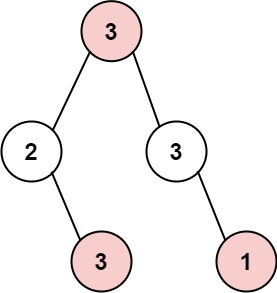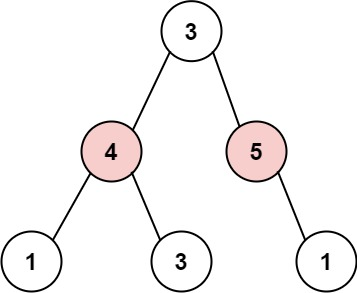## Algorithm

Problem Name: 337. House Robber III

The thief has found himself a new place for his thievery again. There is only one entrance to this area, called `root`.

Besides the `root`, each house has one and only one parent house. After a tour, the smart thief realized that all houses in this place form a binary tree. It will automatically contact the police if two directly-linked houses were broken into on the same night.

Given the `root` of the binary tree, return the maximum amount of money the thief can rob without alerting the police.

Example 1:```Input: root = [3,2,3,null,3,null,1]
Output: 7
Explanation: Maximum amount of money the thief can rob = 3 + 3 + 1 = 7.
```

Example 2:```Input: root = [3,4,5,1,3,null,1]
Output: 9
Explanation: Maximum amount of money the thief can rob = 4 + 5 = 9.
```

Constraints:

• The number of nodes in the tree is in the range `[1, 104]`.
• `0 <= Node.val <= 104`

## Code Examples

### #1 Code Example with C Programming

```Code - C Programming```

``````
int rob(struct TreeNode* root) {
int val, val_next;
if (!root) return 0;
val = root->val;
if (root->left) val += rob(root->left->left) + rob(root->left->right);
if (root->right) val += rob(root->right->left) + rob(root->right->right);
val_next = rob(root->left) + rob(root->right);

return val > val_next ? val : val_next;
}
``````
Copy The Code &

Input

cmd
root = [3,2,3,null,3,null,1]

Output

cmd
7

### #2 Code Example with Java Programming

```Code - Java Programming```

``````
class Solution {
public int rob(TreeNode root) {
int[] ans = helper(root);
return Math.max(ans, ans);
}

private int[] helper(TreeNode root) {
if (root == null) {
return new int[]{0, 0};
}
int[] left = helper(root.left);
int[] right = helper(root.right);
int robbed = root.val + left + right;
int notRobbed = Math.max(left, left) + Math.max(right, right);
return new int[]{robbed, notRobbed};
}
}
``````
Copy The Code &

Input

cmd
root = [3,2,3,null,3,null,1]

Output

cmd
7

### #3 Code Example with Javascript Programming

```Code - Javascript Programming```

``````
const rob = function(root) {
return Math.max(...dfs(root))
}

function dfs(node) {
if (node == null) return [0, 0]
const left = dfs(node.left)
const right = dfs(node.right)
return [
node.val + left + right,
Math.max(left, left) + Math.max(right, right)
]
}
``````
Copy The Code &

Input

cmd
root = [3,4,5,1,3,null,1]

Output

cmd
9

### #4 Code Example with Python Programming

```Code - Python Programming```

``````
class Solution:
def rob(self, root):
def dfs(node):
if not node: return 0, 0
l, r = dfs(node.left), dfs(node.right)
return max(l) + max(r), node.val + l + r
return max(dfs(root))
``````
Copy The Code &

Input

cmd
root = [3,4,5,1,3,null,1]

Output

cmd
9